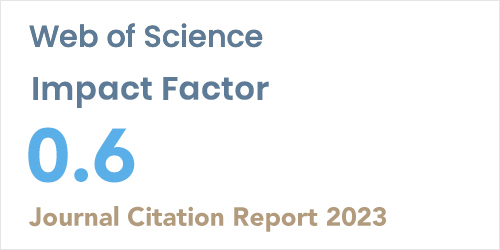ISSN: 1304-7191 | E-ISSN: 1304-7205
Volume: 41 Issue: 4 Year: 2023Optimal Boundary Control for a Second Strain Gradient Theory-Based Beam Model
1Istanbul Gedik University, Department of Computer Engineering, ISTANBUL
2Istanbul Sabahattin Zaim University, Electrical and Electronics Engineering, ISTANBUL
3Mus Alparslan University, Department of Mathematics and Science Education, MUS
Sigma J Eng Nat Sci 2019; 37(4): 1281-1292

#### Abstract

The second strain gradient theory is a non-classical continuum theory that captures the behavior of micrometer and nanometer sized beam structures. Timoshenko and Euler-Bernoulli theories are classical beam models that neglect the effects of small size structures when compared to the second strain gradient theory-based beam model. In this study, an optimal boundary control problem is formulated for the second strain gradient theory-based beam model to control free vibrations in the system. A quadratic performance index expressing the dynamic response of the system is to be minimized while an affordable control is in use. An indirect method based on Pontryagin’s maximum principle is used to derive a necessary condition analytically for optimal control. Then, the problem is transformed into a system of partial differential equations consisting of state and costate (adjoint) variables together. The solution of the control problem is carried out using the computer codes produced in MATLAB©. The effectiveness and competence of the introduced optimal boundary control are presented in numerical simulations.